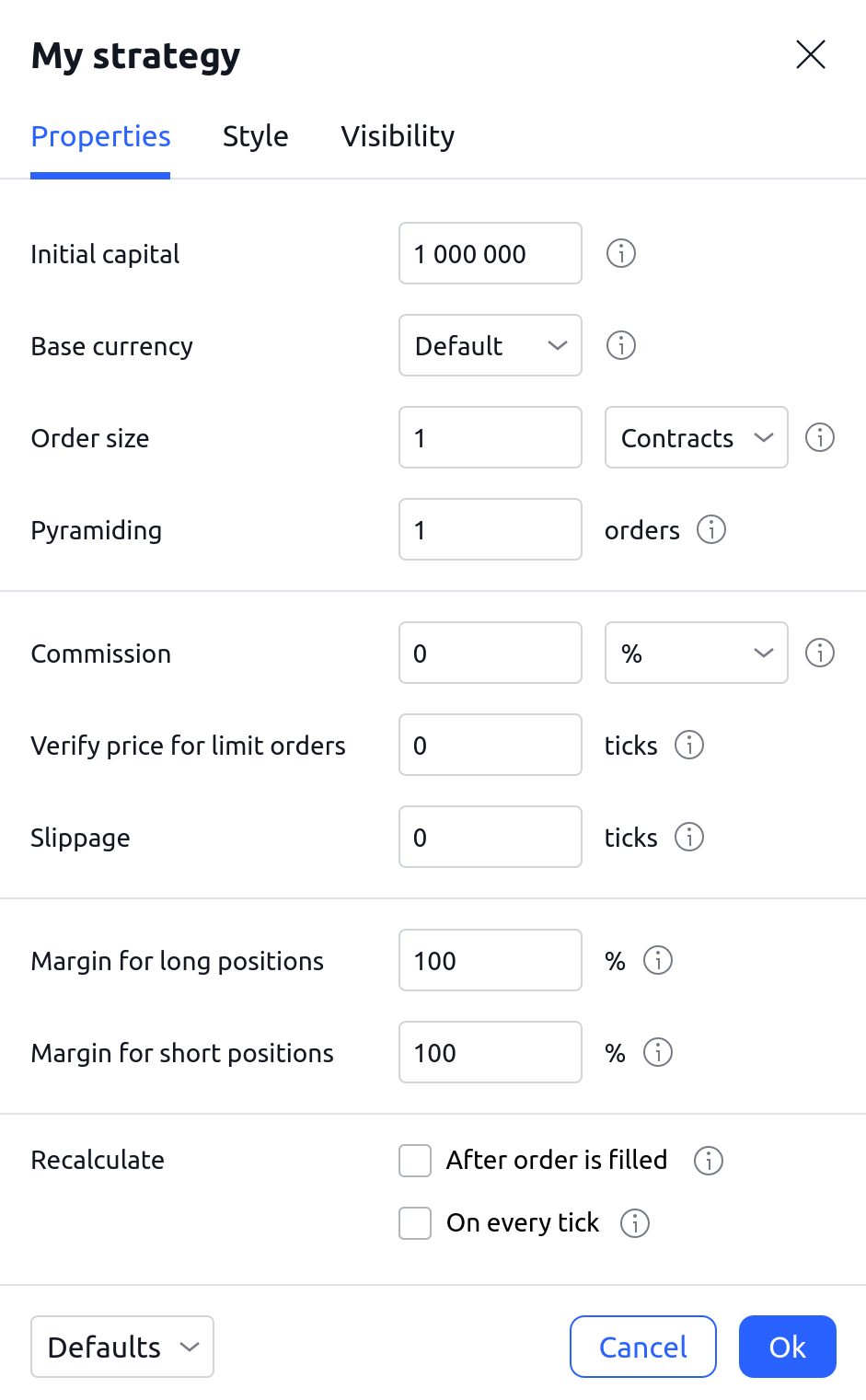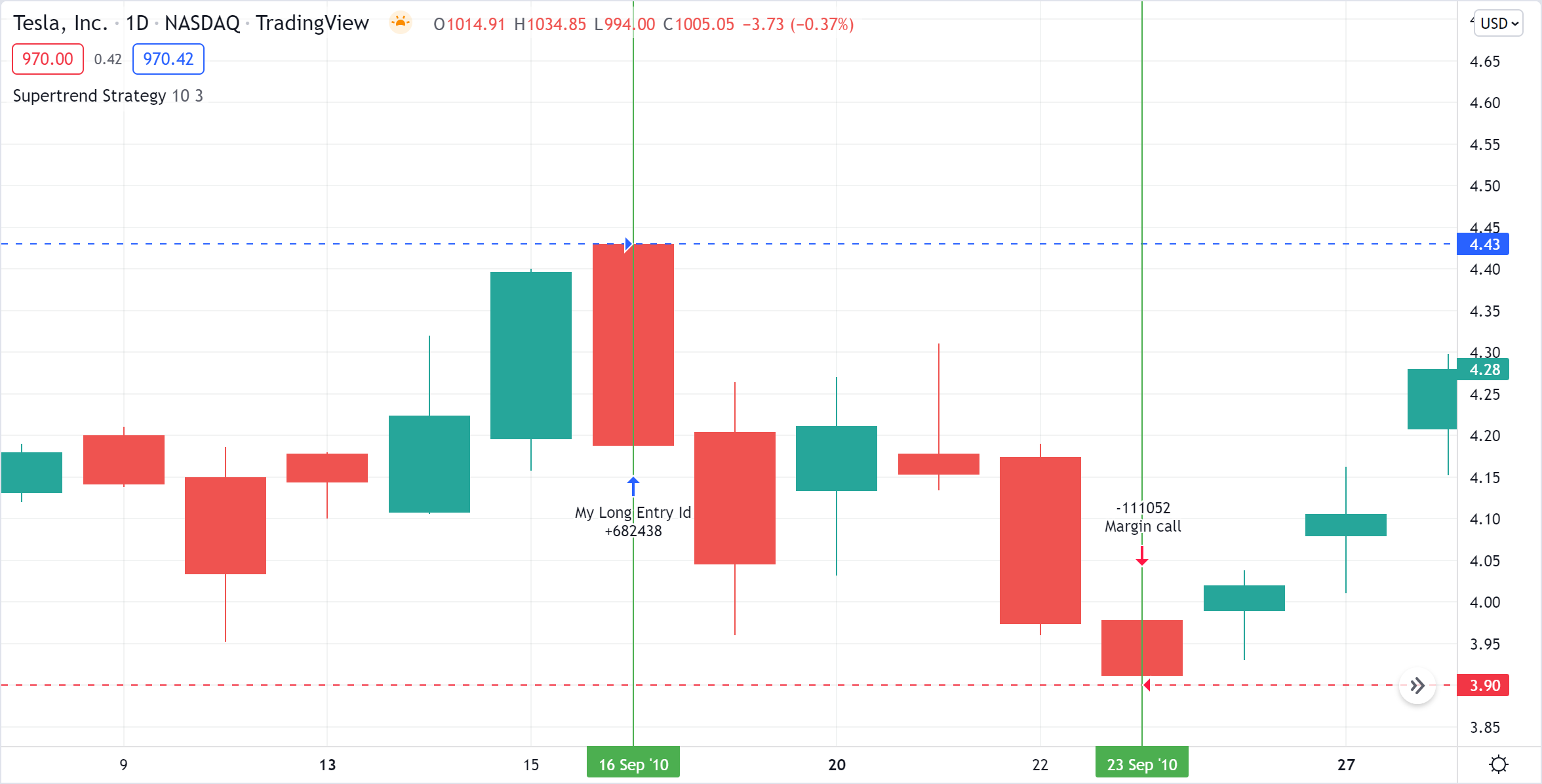# Strategy properties

#### Each Pine strategy has a number of properties that determine its behavior:

1. Initial Capital
2. Base Currency
3. Order Size
4. Pyramiding
5. Commission
6. Verify Price For Limit Orders
7. Slippage
8. Margin
9. Recalculate

They are available in the strategy settings, in the Properties tab:Each of the parameters specified in the properties of the strategy can be changed by editing the arguments of the strategy() function call in the corresponding Pine script:

strategy(title, initial_capital, currency, default_qty_value, default_qty_type, pyramiding, commission_type, commission_value, backtest_fill_limits_assumption, slippage, process_orders_on_close, margin_long, margin_short, calc_on_order_fills, calc_on_every_tick)

Let's take a look at each input parameter in the Properties menu and its corresponding parameter in the Pine language:

1 - Initial Capital (parameter: initial_capital) represents the amount of funds initially available for the strategy to trade, in the currency defined in Base Currency. By default, this value is equal to 1,000,000. You may need to increase this value for trades to occur on certain symbols.

2 - Base Currency (parameter: currency) specifies the currency used for calculations. Results appearing in the Strategy Tester tab (profit, loss, drawdown, etc) are expressed in this currency. Available choices are:

Default, USD, EUR, AUD, GBP, NZD, CAD, CHF, HKD, JPY, NOK, SEK, SGD, TRY, ZAR. If the Default choice is selected, the strategy will use the default currency for this symbol and there is no currency conversion.

3 - Order Size (parameters: default_qty_value, default_qty_type). This requires a value and a calculation mode. Note that the calculated values can be subject to constraints due to the minimum tradable quantities for the symbol:

• Contracts (argument: strategy.fixed) - the strategy will enter with the specified number of contracts/shares/lots.
• Amount in currency (argument: strategy.cash) - the strategy will enter the amount specified in base currency.
• Percentage of equity (argument: strategy.percent_of_equity) - position sizes will be calculated as a percentage of the available equity when the trade opens.

4 - Pyramiding (parameter: pyramiding) specifies the maximum number of successive entries allowed in the same direction. When pyramiding is disabled, the strategy can only open one long or short position, even if entry conditions are met. Pyramiding only affects entries made using the strategy.entry() function. It has no effect on orders created using strategy.order().

5 - Commission (parameters: commission_typecommission_value). It is the amount paid in trading fees for each trade. A value and calculation mode must be supplied. Note that commission is applied on both entries and exits, and that when a percentage is used, the calculated commission will vary with the value of the transaction:

• Percentage of the transacted value (argument: strategy.commission.percent) - imposes a commission on each order equal to the specified percentage.
• Currency per contract (argument: strategy.commission.cash_per_contract) - imposes a commission on each contract.
• Currency per order (argument: strategy.commission.cash_per_order) - imposes a commission on each order.

6 - Verify Price For Limit Orders (parameter: backtest_fill_limits_assumption) makes the conditions for entering a position using limit orders more strict. By default, this value is 0, i.e. limit orders are filled on historical data as soon as the price indicated in the order is reached. If the parameter is not zero, then limit orders can enter a position within the bar only if the market price has exceeded the level of the limit order by the specified number of ticks.

7 - Slippage (parameter: slippage) specifies the value in ticks to be added to the fill price of market or stop orders. It can be used to account for the spread.

8 - Margin for Long/Short positions (parameters: margin_long, margin_short) specifies the margin for each trade, i.e., the percent of the position that the trader must fund. For example, if the Margin for long positions is set to 25%, the trader has to have enough funds to cover 25% of the open trade and can potentially spend up to 400% of their equity on every trade.

If a trade has been opened and it starts losing money to the extent where the trader's funds are not enough to cover their portion of the trade, a Margin Call occurs and forcibly liquidates a part of the original position. The precise number of units that will be liquidated is 4 times the amount it takes to simply cover the loss. It is calculated via the following algorithm:

1. Calculate Money Spent, the amount of money the trader has spent on opening the position.

Position Size * Entry Price

2. Calculate the Market Value of Security (MVS).

Position Size * Current Price

3. Calculate the Open Profit. If the trade direction is short and Open Profit is a positive number, the result should still be negative, so we multiply the absolute value of our calculation by -1.

ABS(MVS - Money Spent) * -1

4. Calculate the Equity, i.e., the money available to the trader at the current moment.

Initial Capital + Net Profit + Open Profit

5. Convert Margin Percent to Margin Ratio.

Margin Percent / 100

6. Calculate Margin, i.e., the exact amount of money needed to cover their part of the open position.

MVS * Margin Ratio

7. Calculate Available Funds, i.e., the amount of lost money the trader cannot cover with their current equity.

Equity - Margin

8. Calculate the total amount of money the trader has lost.

Available Funds / Margin Ratio

9. Calculate how many units the trader would need to sell to cover the loss. The value is truncated to the same decimal point as the minimum contract size for the current symbol.

TRUNCATE(Step #8 / Current Price)

10. Calculate how many units the broker will sell to cover the loss. Our emulated broker sells 4 times as many units as necessary to make sure the margin call isn't constantly triggered if the losses continue. This value will be positive for short trades because the broker buys units to cover the loss instead of selling them.

Step #9 * 4

To examine this calculation in detail, let's add the built-in Supertrend Strategy to the NASDAQ:TSLA chart on the 1D timeframe. Set Order size to 300% of equity and Margin for long positions to 25%.Our first entry happened on the opening of the bar on 16 Sep 2010. We buy 682438 units (Position size) for 4.43 USD (Entry price). Then, on 23 Sep 2010, when the price was at 3.9 (Current price), 111052 units were forcibly liquidated via margin call.

1. Money spent: 682438 * 4.43 = 3023200.34

2. MVS: 682438 * 3.9 = 2661508.2

3. Open Profit: −361692.14

4. Equity: 1000000 + 0 − 361692.14 = 638307.86

5. Margin Ratio: 25 / 100 = 0.25

6. Margin: 2661508.2 * 0.25 = 665377.05

7. Available Funds: 638307.86 - 665377.05 = -27069.19

8. Money Lost: -27069.19 / 0.25 = -108276.76

9. Shares to cover the loss: TRUNCATE(-108276.76 / 3.9) = TRUNCATE(-27763.27) = -27763

10. Margin Call Size: -27763 * 4 = - 111052

9 - Recalculate options specify how often the strategy should be recalculated. By default, the strategy is recalculated at the close of each bar, but using the options below, it can also be recalculated:

• After Order is Filled (parameter: calc_on_order_fills) - allows the strategy to perform an additional intra-bar order calculation immediately after an order fills. That extra calculation happens on both historical and realtime bars.
• On Every Tick (parameter: calc_on_every_tick). By default, strategies only calculate on the close of realtime bars. This parameter allows the strategy to calculate on each update of realtime bars, like an indicator would. Note that tick data is lost when the chart is refreshed, so strategies using this option will repaint. This parameter does not affect the behavior of strategies on historical bars. Also note that strategies using this feature will not show realistic results on historical bars, as they contain no tick data.